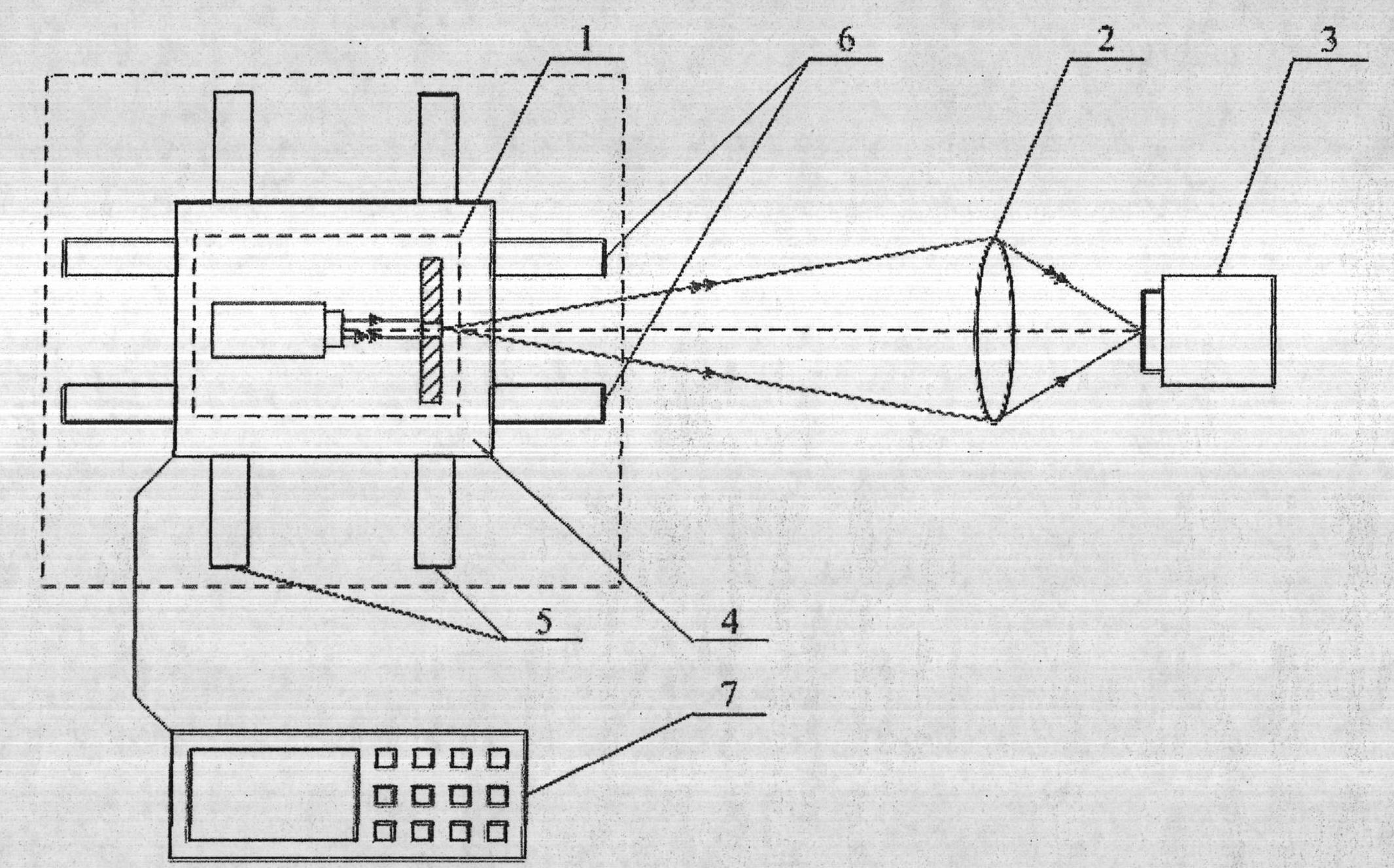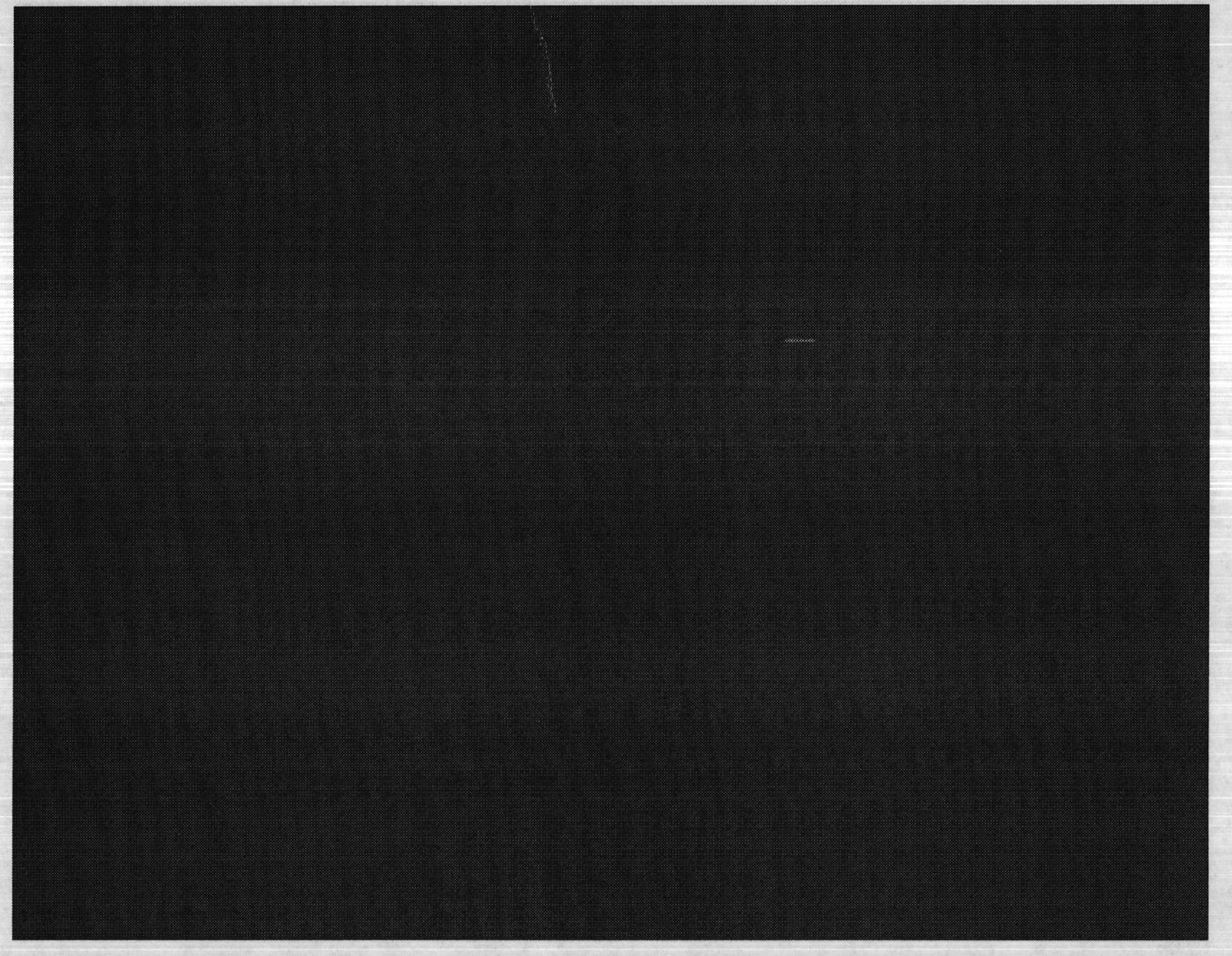# Method and device for measuring transverse magnification of optical system by means of uniform-speed moving point targets

## A technology of lateral magnification and optical system, which is applied in the field of lateral magnification measurement and device of optical system using a uniform moving point target, can solve the problems of low repeatability of lateral magnification measurement, achieve small errors, reduce errors, and improve repetitive effect

Active Publication Date: 2012-08-01
HARBIN INST OF TECH
9 Cites 8 Cited by

## AI-Extracted Technical Summary

### Problems solved by technology

 The present invention aims at the large distortion optical system of the above-mentioned existing measurement method, which is not suitable for measurement in a large field of view, but in a small field of view, there is the problem of low repeatability of lateral magnification measurement, and the existing measurement device has the problem of separation In view of the problem of focusing, a metho...
the structure of the environmentally friendly knitted fabric provided by the present invention; figure 2 Flow chart of the yarn wrapping machine for environmentally friendly knitted fabrics and storage devices; image 3 Is the parameter map of the yarn covering machine
View more

## Abstract

A method and a device for measuring transverse magnification of an optical system by means of uniform-speed moving point targets belong to the field of metering equipment characterized by an optical method. The method includes: imaging point targets moving at a uniform speed to obtain a linear image; finding the value range of pixel spacing in a frequency domain; and calculating the transverse magnification of the optical system by means of genetic algorithm according to the fact that a practical modulation transfer function curve and a theoretic modulation transfer function curve related to the pixel spacing have the highest overlap ratio under the least square condition. In the device, sliders for bearing the point targets are mounted on a first guide rail and a second guide rail, a controller controls the sliders to move on the first guide rail at a uniform speed while controlling the sliders to move on the second guide rail, and movements in two directions are matched with each other, so that the point targets are constantly focused and imaged on the surface of an image sensor during movement. By the aid of the method and the device for measuring the transverse magnification of the optical system, errors of single measuring results can be decreased, and further repeatability of measuring results is improved.

Application Domain

Technology Topic

## Image

•••## Examples

• Experimental program(1)

### Example Embodiment

 Specific embodiments of the present invention will be further described in detail below in conjunction with the accompanying drawings.
 figure 1 It is a structural schematic diagram of an optical system lateral magnification measurement device using a uniform moving point target; the device includes a point target 1, an optical system 2, an image sensor 3, a slider 4, a first guide rail 5 perpendicular to the optical axis direction, and a controller 7, The point target 1 is imaged onto the surface of the image sensor 3 through the optical system 2; and, the device also includes a second guide rail 6 along the optical axis direction, and the slider 4 carrying the point target 1 is installed on the first guide rail 5 and the second guide rail 5. On the guide rail 6, when the controller 7 controls the slider 4 to move at a constant speed on the first guide rail 5, the controller 7 controls the slider 4 to move on the second guide rail 6, and the movement in the two directions cooperates to make the point target 1 in During the movement, images are always in-focus on the surface of the image sensor 3; the point target 1 is a pinhole with a diameter of 15 μm, and the pixel pitch of the image sensor 3 is 5.6 μm.
 The method for measuring the lateral magnification of the optical system using a uniform moving point target, the flow chart is as follows figure 2 As shown, the method steps are as follows:
 a. Set the moving speed v=8.5mm/s of the point target 1 and the exposure time t=359ms of the image sensor 3, the movement displacement of the point target 1 on the object side can be calculated as: d=v·t=8.5×359× 10 -3 = 3.0515mm;
 b. Under the parameters in step a, the image sensor 3 images the point target 1 moving along the direction of the image sensor 3 lines, and obtains the initial point spread function image, such as image 3. Shown; Keep the exposure time t=359ms of the image sensor 3 unchanged, remove the point target 1, the image sensor 3 images the background, obtains the interference image, and uses the maximum value of the gray value in the interference image as the threshold, the threshold is 10;
 c. From the initial point spread function image obtained in step b, extract the entire line information of the point target 1 swept line as the initial line spread function image, such as Figure 4 As shown, and the gray value of the pixel whose gray value in the initial line spread function image is smaller than the threshold value obtained in step b is corrected to 0, and the corrected line spread function image is obtained, as shown in Figure 5 As shown, the modified line extension function image has n=1280 elements;
 or:
 In the initial point spread function image obtained in step b, the gray value of the pixel whose gray value is smaller than the threshold obtained in step b is corrected to 0, as the corrected point spread function image; and in the corrected point spread function image, the point target The entire line information of the scanned line is extracted as a correction line extension function image, such as Figure 5 As shown, the modified line extension function image has n=1280 elements;
 d. Discrete Fourier transform and modulus are performed on the modified line spread function image obtained in the c step to obtain a modulation transfer function image, which has the same number of elements as the modified line spread function image obtained in the c step Number n=1280, that is, 1280 discrete spectral components, according to the order of spatial frequency from small to large, respectively M 0 , M 1 , M 2 ,...,M 1279 , in this order, the MTF value corresponding to the MTF value reaching the minimum value for the first time is M 42 , its subscript sequence number is i=42, combined with the pixel pitch l=5.6 μm of the image sensor 3, M 41 and M 43 The corresponding spatial frequency values ​​are: f min =(i-1)/(nl)=(42-1)/(1280×5.6×10 -3 )=5.7199lp/mm and f max =(i+1)/(nl)=(42+1)/(1280×5.6×10 -3 ) = 5.9989lp/mm;
 e. According to the modulation transfer function model MTF(f)=|sinc(πfd')|, combined with the spatial frequency range f obtained in step d min = 5.7199 lp/mm and f max =5.9989lp/mm, the value range of the motion displacement of point target 1 in the image space is obtained: d max '=1/f min =nl/(i-1)=1280×5.6×10 -3 /(42-1)=0.1748mm and d min '=1/f max =nl/(i+1)=1280×5.6×10 -3 /(42+1)=0.1667mm;
 f. According to the motion displacement d=3.0515mm of point target 1 in the object space obtained in step a and the value range d of the motion displacement of point target 1 in image space obtained in step e min '=0.1667mm and d max ′=0.1748mm, the calculated value range of the lateral magnification of the optical system 3 is: β min = d min '/d=nl/((i+1)d)=1280×5.6×10 -3 /((42+1)×3.0515)=0.0546 and β max = d max '/d=nl/((i-1)d)=1280×5.6×10 -3 /((42-1)×3.0515)=0.0573;
 g. Take β as a variable, and use the β obtained in step f min = 0.0546 and β max =0.0573 is the value range, according to the order of spatial frequency from small to large, the n=1280 modulation transfer function values ​​obtained in step d are drawn into a curve, and the curve is selected from M 0 Start to the first maximum value, and do not include the M obtained in the dth step 42 , a total of K as comparison data, the K modulation transfer function values ​​are M K1, M K2 ,...,M KK , using the genetic algorithm to find the minimum value of the following formula: The lateral magnification β of the optical system 3 corresponding to the minimum value is the desired value, and β=0.0558 through calculation.
the structure of the environmentally friendly knitted fabric provided by the present invention; figure 2 Flow chart of the yarn wrapping machine for environmentally friendly knitted fabrics and storage devices; image 3 Is the parameter map of the yarn covering machine

## PUM## Description & Claims & Application Information

We can also present the details of the Description, Claims and Application information to help users get a comprehensive understanding of the technical details of the patent, such as background art, summary of invention, brief description of drawings, description of embodiments, and other original content. On the other hand, users can also determine the specific scope of protection of the technology through the list of claims; as well as understand the changes in the life cycle of the technology with the presentation of the patent timeline. Login to view more.
the structure of the environmentally friendly knitted fabric provided by the present invention; figure 2 Flow chart of the yarn wrapping machine for environmentally friendly knitted fabrics and storage devices; image 3 Is the parameter map of the yarn covering machine

## Method and device for measuring lateral magnification of optical system

Owner:HARBIN INST OF TECH

## Lateral magnification measuring method for point target image-spliced optical system and lateral magnification measuring device

Owner:HARBIN INST OF TECH

## Lentinan molecular weight and molecular weight distribution measuring method

Owner:NANJING LUYE PHARMA

## Method and device for measuring optical system lateral magnification by utilizing uniform-speed moving point target

Owner:HARBIN INST OF TECH

## Method and device for measuring pixel pitch of image sensor based on point-target image splicing technology

Owner:HARBIN INST OF TECH

## Classification and recommendation of technical efficacy words

• Amortized error
• Reduce mistakes

## Lateral magnification measuring method for point target image-spliced optical system and lateral magnification measuring device

Owner:HARBIN INST OF TECH

## Method and device for measuring pixel pitch of image sensor based on point-target image splicing technology

Owner:HARBIN INST OF TECH

## Method and device for measuring optical system lateral magnification by utilizing uniform-speed moving point target

Owner:HARBIN INST OF TECH

## Method and device for measuring lateral magnification of optical system

Owner:HARBIN INST OF TECH
Who we serve
• R&D Engineer
• R&D Manager
• IP Professional
Why Eureka